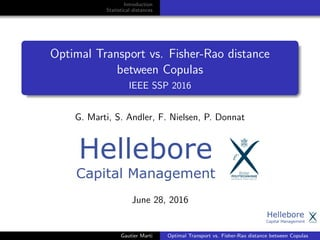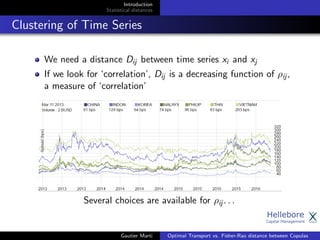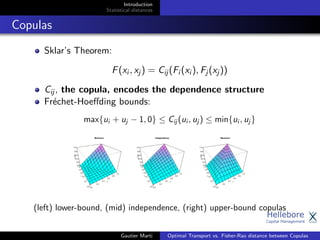Successfully reported this slideshow.

# Optimal Transport vs. Fisher-Rao distance between Copulas

2

Share×
1 of 18
1 of 18

# Optimal Transport vs. Fisher-Rao distance between Copulas

2

Share

How can we compare two dependence structures (represented by copulas)? It depends on the task. For clustering variables with similar dependence, prefer Optimal Transport. For detecting change points in a dynamical dependence structure, prefer Fisher-Rao and its associated f-divergences (for example, an approach a la Frédéric Barbaresco in radar signal processing). This study illustrates these properties with bivariate Gaussian copulas.

How can we compare two dependence structures (represented by copulas)? It depends on the task. For clustering variables with similar dependence, prefer Optimal Transport. For detecting change points in a dynamical dependence structure, prefer Fisher-Rao and its associated f-divergences (for example, an approach a la Frédéric Barbaresco in radar signal processing). This study illustrates these properties with bivariate Gaussian copulas.

## More Related Content

### Related Books

Free with a 14 day trial from Scribd

See all

### Related Audiobooks

Free with a 14 day trial from Scribd

See all

### Optimal Transport vs. Fisher-Rao distance between Copulas

1. 1. Introduction Statistical distances Optimal Transport vs. Fisher-Rao distance between Copulas IEEE SSP 2016 G. Marti, S. Andler, F. Nielsen, P. Donnat June 28, 2016 Gautier Marti Optimal Transport vs. Fisher-Rao distance between Copulas
2. 2. Introduction Statistical distances Clustering of Time Series We need a distance Dij between time series xi and xj If we look for ‘correlation’, Dij is a decreasing function of ρij , a measure of ‘correlation’ Several choices are available for ρij . . . Gautier Marti Optimal Transport vs. Fisher-Rao distance between Copulas
3. 3. Introduction Statistical distances Copulas Sklar’s Theorem: F(xi , xj ) = Cij (Fi (xi ), Fj (xj )) Cij , the copula, encodes the dependence structure Fr´echet-Hoeﬀding bounds: max{ui + uj − 1, 0} ≤ Cij (ui , uj ) ≤ min{ui , uj } (left) lower-bound, (mid) independence, (right) upper-bound copulas Gautier Marti Optimal Transport vs. Fisher-Rao distance between Copulas
4. 4. Introduction Statistical distances Copulas - Gaussian Example Gaussian copula: CGauss R (ui , uj ) = ΦR(Φ−1(ui ), Φ−1(uj )) The distribution is parametrized by a correlation matrix R. Gautier Marti Optimal Transport vs. Fisher-Rao distance between Copulas
5. 5. Introduction Statistical distances The Target/Forget (copula-based) Dependence Coeﬃcient Dependence is measured as the relative distance from independence to the nearest target-dependence: comonotonicity or counter-monotonicity Which distances are appropriate between copulas for the task of clustering (copulas and time series)? Gautier Marti Optimal Transport vs. Fisher-Rao distance between Copulas
6. 6. Introduction Statistical distances Deﬁnitions - Fisher-Rao geodesic distance Metrization of the paramater space {θ ∈ Rd | p(X; θ)dx = 1}. Consider the metric gjk(θ) = − ∂2 log p(x,θ) ∂θj ∂θk p(x, θ)dx, the inﬁnitesimal length ds(θ) = ( θ) G(θ) θ, the Fisher-Rao geodesic distance FR(θ1, θ2) = θ2 θ1 ds(θ). f -divergences induce inﬁnitesimal length proportional to Fisher-Rao inﬁnitesimal length: Df (θ θ + dθ) = 1 2 ( θ) G(θ) θ. Thus, they have the same local behaviour . Gautier Marti Optimal Transport vs. Fisher-Rao distance between Copulas
7. 7. Introduction Statistical distances Deﬁnitions - Optimal Transport distances Wasserstein metric Wp(µ, ν)p = inf γ∈Γ(µ,ν) M×M d(x, y)p dγ(x, y) Image from Optimal Transport for Image Processing, Papadakis Other transportation distances: regularized discrete optimal transport , Sinkhorn distances , . . . Gautier Marti Optimal Transport vs. Fisher-Rao distance between Copulas
8. 8. Introduction Statistical distances Geometry of covariances Gautier Marti Optimal Transport vs. Fisher-Rao distance between Copulas
9. 9. Introduction Statistical distances Distances between Gaussian copulas Copulas C1, C2, C3 encoding a correlation of 0.5, 0.99, 0.9999 respectively; Which pair of copulas is the nearest? - For Fisher-Rao, Kullback-Leibler, Hellinger and related divergences: D(C1, C2) ≤ D(C2, C3); - For Wasserstein: W2(C2, C3) ≤ W2(C1, C2) Gautier Marti Optimal Transport vs. Fisher-Rao distance between Copulas
10. 10. Introduction Statistical distances Distances as a function of (ρ1, ρ2) Distance heatmap and surface as a function of (ρ1, ρ2) for Fisher-Rao for Wasserstein W2 Gautier Marti Optimal Transport vs. Fisher-Rao distance between Copulas
11. 11. Introduction Statistical distances Distances impact on clustering Datasets of bivariate time series are generated from six Gaussian copulas with correlation .1, .2, .6, .7, .99, .9999 Distance heatmaps for Fisher-Rao (left), W2 (right); Using Ward clustering, Fisher-Rao yields clusters of copulas with correlations {.1, .2, .6, .7}, {.99}, {.9999}, W2 yields {.1, .2}, {.6, .7}, {.99, .9999} Gautier Marti Optimal Transport vs. Fisher-Rao distance between Copulas
12. 12. Introduction Statistical distances Fisher metric and the Cram´er–Rao lower bound Cram´er–Rao lower bound (CRLB) The variance of any unbiased estimator ˆθ of θ is bounded by the reciprocal of the Fisher information G(θ): var(ˆθ) ≥ 1 G(θ) . In the bivariate Gaussian copula case, var(ˆρ) ≥ (ρ − 1)2(ρ + 1)2 3(ρ2 + 1) . Gautier Marti Optimal Transport vs. Fisher-Rao distance between Copulas
13. 13. Introduction Statistical distances Fisher metric and the Cram´er–Rao lower bound We consider the set of 2 × 2 correlation matrices C = 1 θ θ 1 parameterized by θ. Let x = x1 x2 ∈ R2 . f (x; θ) = 1 2π 1−θ2 exp − 1 2 x C−1 x = 1 2π 1−θ2 exp − 1 2(1−θ2) (x2 1 + x2 2 − 2θx1x2) log f (x; θ) = − log(2π 1 − θ2) − 1 2(1−θ2) (x2 1 + x2 2 − 2θx1x2) ∂2 log f (x;θ) ∂θ2 = − θ2+1 (θ2−1)2 − x2 1 2(θ+1)3 + x2 1 2(θ−1)3 − x2 2 2(θ+1)3 + x2 2 2(θ−1)3 − x1x2 (θ+1)3 − x1x2 (θ−1)3 Then, we compute ∞ −∞ ∂2 log f (x;θ) ∂θ2 f (x; θ)dx. Since E[x1] = E[x2] = 0, E[x1x2] = θ, E[x2 1 ] = E[x2 2 ] = 1, we get ∞ −∞ ∂2 log f (x;θ) ∂θ2 f (x; θ)dx = − θ2+1 (θ2−1)2 − 1 2(θ+1)3 + 1 2(θ−1)3 − 1 2(θ+1)3 + 1 2(θ−1)3 − θ (θ+1)3 − θ (θ−1)3 = − 3(θ2+1) (θ−1)2(θ+1)2 Thus, G(θ) = 3(θ2 + 1) (θ − 1)2(θ + 1)2 . Gautier Marti Optimal Transport vs. Fisher-Rao distance between Copulas
14. 14. Introduction Statistical distances Fisher metric and the Cram´er–Rao lower bound In the bivariate Gaussian copula case, var(ˆρ) ≥ (ρ − 1)2(ρ + 1)2 3(ρ2 + 1) . Recall that locally Fisher-Rao and the f -divergences are a quadratic form of the Fisher metric ( θ) G(θ) θ. So, the discriminative power of these distances is well calibrated with respect to statistical uncertainty. For this purpose, they induce the appropriate curvature on the parameter space. Gautier Marti Optimal Transport vs. Fisher-Rao distance between Copulas
15. 15. Introduction Statistical distances Properties of these distances In addition, for clustering we prefer OT since: in a parametric setting: Fisher-Rao and f -divergences are deﬁned on density manifolds, but some important copulas (such as the Fr´echet-Hoeﬀding upper bound) do not belong to these manifolds; Thus, in case of closed-form formulas (such as in the Gaussian case), they are ill-deﬁned for these copulas (for perfect dependence, covariance is not invertible) in a non-parametric/empirical setting: f -divergences are deﬁned for absolutely continuous measures, thus require a pre-processing KDE they are not aware of the support geometry, thus badly handle noise on the support Gautier Marti Optimal Transport vs. Fisher-Rao distance between Copulas
16. 16. Introduction Statistical distances Barycenters OT is deﬁned for both discrete/empirical and continuous measures and is support-geometry aware: 0 0.5 1 0 0.5 1 0.0000 0.0015 0.0030 0.0045 0.0060 0.0075 0.0090 0.0105 0.0120 0 0.5 1 0 0.5 1 0.0000 0.0015 0.0030 0.0045 0.0060 0.0075 0.0090 0.0105 0.0120 0 0.5 1 0 0.5 1 0.0000 0.0008 0.0016 0.0024 0.0032 0.0040 0.0048 0.0056 0 0.5 1 0 0.5 1 0.0000 0.0015 0.0030 0.0045 0.0060 0.0075 0.0090 0.0105 0.0120 0 0.5 1 0 0.5 1 0.0000 0.0015 0.0030 0.0045 0.0060 0.0075 0.0090 0.0105 0.0120 5 copulas describing the dependence between X ∼ U([0, 1]) and Y ∼ (X ± i )2 , where i is a constant noise speciﬁc for each distribution 0 0.5 1 0 0.5 1 Wasserstein barycenter copula 0.0000 0.0004 0.0008 0.0012 0.0016 0.0020 0.0024 0.0028 0.0032 Barycenter of the 5 copulas for a divergence and OT Gautier Marti Optimal Transport vs. Fisher-Rao distance between Copulas
17. 17. Introduction Statistical distances Future Research Develop further geometries of copulas using Optimal Transport: show that dependence-clustering of time series is improved over standard correlations using f -divergences: detect eﬃciently dependence-regime switching in multivariate time series (cf. Fr´ed´eric Barbaresco’s work on radar signal processing) Numerical experiments and code: https://www.datagrapple.com/Tech/fisher-vs-ot.html Gautier Marti Optimal Transport vs. Fisher-Rao distance between Copulas
18. 18. Introduction Statistical distances Shun-ichi Amari and Andrzej Cichocki. Information geometry of divergence functions. Bulletin of the Polish Academy of Sciences: Technical Sciences, 58(1):183–195, 2010. Marco Cuturi. Sinkhorn distances: Lightspeed computation of optimal transport. In Advances in Neural Information Processing Systems, pages 2292–2300, 2013. Sira Ferradans, Nicolas Papadakis, Julien Rabin, Gabriel Peyr´e, and Jean-Fran¸cois Aujol. Regularized discrete optimal transport. Springer, 2013. Gautier Marti Optimal Transport vs. Fisher-Rao distance between Copulas